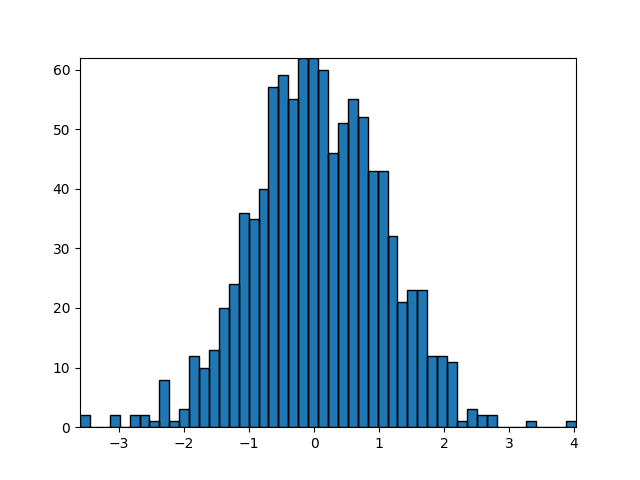# Building histograms using Rectangles and PolyCollections¶

Using a path patch to draw rectangles. The technique of using lots of Rectangle instances, or the faster method of using PolyCollections, were implemented before we had proper paths with moveto/lineto, closepoly etc in mpl. Now that we have them, we can draw collections of regularly shaped objects with homogeneous properties more efficiently with a PathCollection. This example makes a histogram – its more work to set up the vertex arrays at the outset, but it should be much faster for large numbers of objects```import numpy as np
import matplotlib.pyplot as plt
import matplotlib.patches as patches
import matplotlib.path as path

fig, ax = plt.subplots()

# Fixing random state for reproducibility
np.random.seed(19680801)

# histogram our data with numpy

data = np.random.randn(1000)
n, bins = np.histogram(data, 50)

# get the corners of the rectangles for the histogram
left = np.array(bins[:-1])
right = np.array(bins[1:])
bottom = np.zeros(len(left))
top = bottom + n

# we need a (numrects x numsides x 2) numpy array for the path helper
# function to build a compound path
XY = np.array([[left, left, right, right], [bottom, top, top, bottom]]).T

# get the Path object
barpath = path.Path.make_compound_path_from_polys(XY)

# make a patch out of it
patch = patches.PathPatch(barpath)